# Simulate from Existing Data

#### 2020-09-21

library(ggplot2)
library(dplyr)
library(tidyr)
library(faux)

The sim_df() function produces a dataframe with the same distributions and correlations as an existing dataframe. It only returns numeric columns and simulates all numeric variables from a continuous normal distribution (for now).

For example, here is the relationship between speed and distance in the built-in dataset cars.

cars %>%
ggplot(aes(speed, dist)) +
geom_point() +
geom_smooth(method = "lm", formula = "y~x")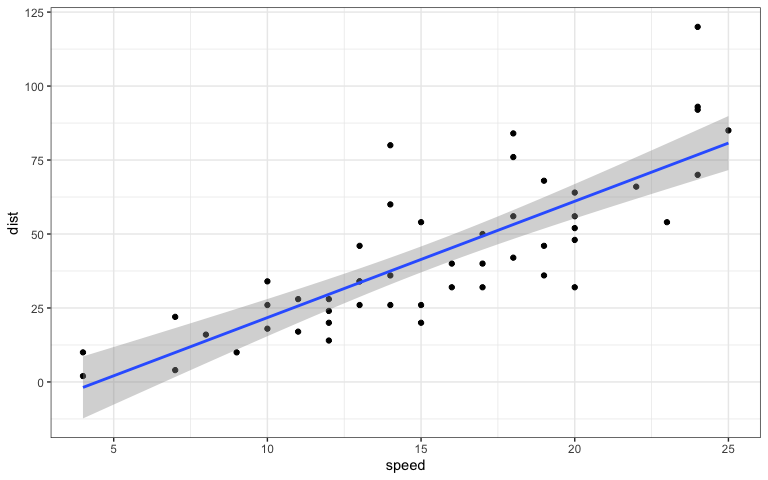Original cars dataset

You can create a new sample with the same parameters and 500 rows with the code sim_df(cars, 500).

sim_df(cars, 500) %>%
ggplot(aes(speed, dist)) +
geom_point() +
geom_smooth(method = "lm", formula = "y~x")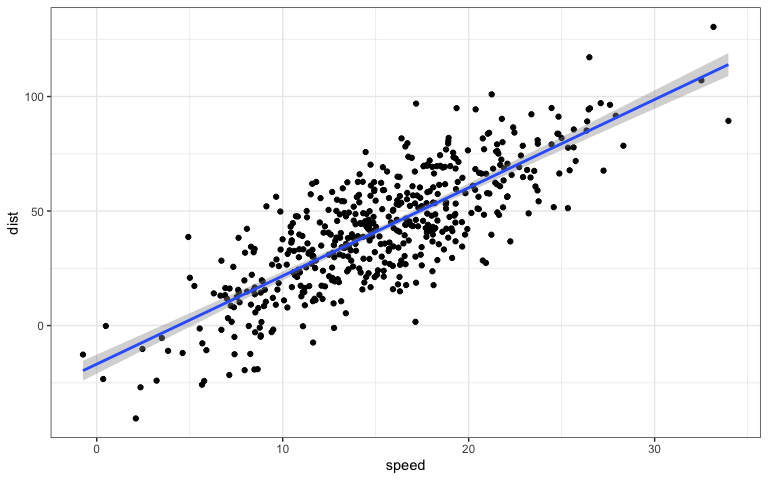Simulated cars dataset

## Between-subject variables

You can also optionally add between-subject variables. For example, here is the relationship between horsepower (hp) and weight (wt) for automatic (am = 0) versus manual (am = 1) transmission in the built-in dataset mtcars.

mtcars %>%
mutate(transmission = factor(am, labels = c("automatic", "manual"))) %>%
ggplot(aes(hp, wt, color = transmission)) +
geom_point() +
geom_smooth(method = "lm", formula = "y~x")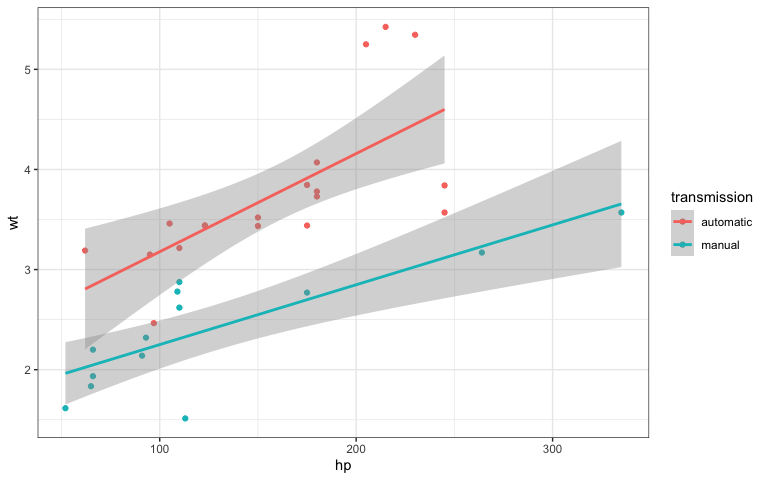Original mtcars dataset

And here is a new sample with 50 observations of each.

sim_df(mtcars, 50 , between = "am") %>%
mutate(transmission = factor(am, labels = c("automatic", "manual"))) %>%
ggplot(aes(hp, wt, color = transmission)) +
geom_point() +
geom_smooth(method = "lm", formula = "y~x")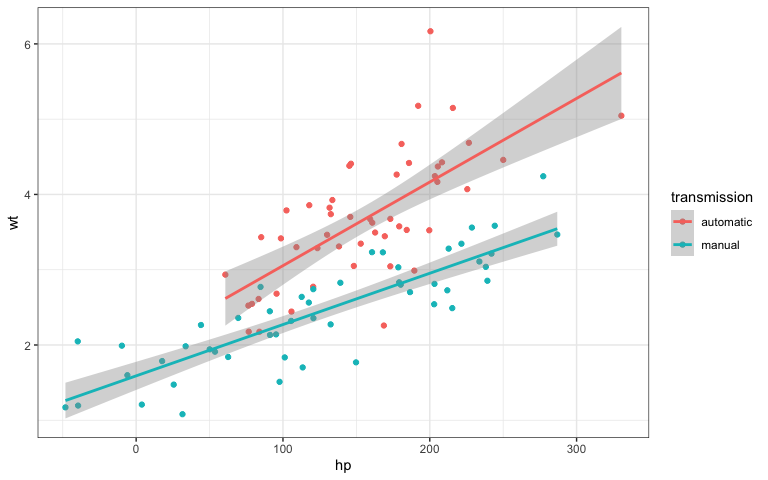Simulated iris dataset

## Empirical

Set empirical = TRUE to return a data frame with exactly the same means, SDs, and correlations as the original dataset.

exact_mtcars <- sim_df(mtcars, 50, between = "am", empirical = TRUE)

## Rounding

For now, the function only creates new variables sampled from a continuous normal distribution. I hope to add in other sampling distributions in the future. So you’d need to do any rounding or truncating yourself.

sim_df(mtcars, 50, between = "am") %>%
mutate(hp = round(hp),
transmission = factor(am, labels = c("automatic", "manual"))) %>%
ggplot(aes(hp, wt, color = transmission)) +
geom_point() +
geom_smooth(method = "lm", formula = "y~x")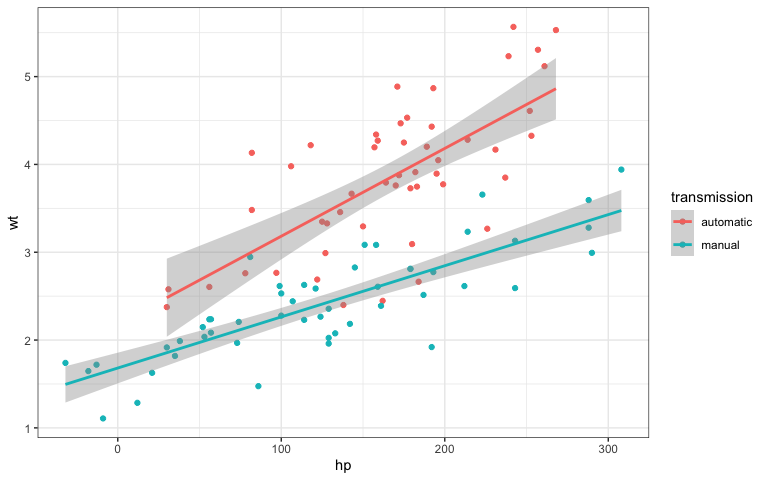Simulated iris dataset (rounded)